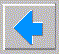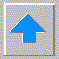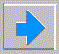# Lecture 6 - Propositional Logic

• Knowledge based agents (RN s6.1)
• more efficient than search since can reason
• KB collection of facts, usually stored as sentences or tables
• Tell, Ask primitives, how does Ask know answers? logical inference
• Example - Wumpus world (RN s6.2)
• Logic (RN s6.3)
• syntax (grammar)
• semantics (meaning)
• correct syntax but not semantics: The smart guitar ran down the cloud.
• inference procedure (proof method)
• Mechanization of logic
• rewrite systems
• syntactical PR
• Rewrite system example
```  1) A is valid (ie. wff and true)
2) if x, y are valid then xy is
3) [a]xx[b] is valid  iff  [a][b] is
4) [a]IAI[b] is valid  iff [a][b] is
5) [a]xy[b] is valid  iff [a]yx[b] is
```
• Question: is AI valid?
• Forward chaining derivations
• A → AA → AAA → AAAA → etc.
• A → AIAI → AAII → II
• A → AIIA → AIIAAIIA → AIAIAIAI
• Backward chaining attempts
• AI ← A and I - but is I a wff?
• AI ← AIIAI ← AAI - but is AAI a wff?
• AI ← AIAIAI - but is AIAIAI a wff ?
• didn't work since all rules lead to an even number of Is.
• jumping out of system; meta-rules
• Propositional logic
• vs. predicate logic, probabilistic logic, fuzzy logic. etc.
• syntax elements: constants, dummy variables, not and or if iff, parentheses
• can only check veracity of proposition - tautology or satisfiability
• syntax
• BNF metalanguages
• ```       sentence ::= atom | complex_sentence
atom ::= true, false, P, Q, ...   (variables)
complex_sentence ::= (sentence) | - sentence | sentence connective sentence
connective ::= and or if iff
```
• examples of wffs and non-wffs
• semantics
• true = anything which exists in the world = environment
• false = opposite of true
• P, Q any statement about environment that has true/false answer
• and, or, if, iff (xor) meanings, show if, iff, etc in terms of and/or/not
• 4 possible 1-input/1-output functions, only not nontrivial, truth tables
• 16 possible 2-input/2-output functions (connectives)
• sufficiency - not+and+or, not+and, not+or, nand, nor
• parentheses and conventions
• cnf, dnf, Horn forms (expert systems)
• systems equivalent to logic
• boolean variables in GF2 (and thus computer software)
• set theory and Venn diagrams
• electronics, eg. relays (and thus computer hardware)
• Wumpus world (RN s6.2)
• Decidability
• truth tables
• complexity - 3SAT is NP-complete
• Simplification inference rules
• De Morgan rules